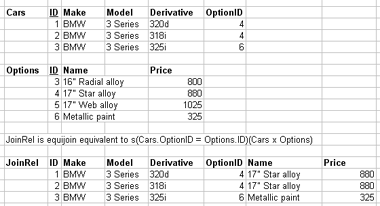# 1.3.10. Join types (condition)

• Theta: Ai q Bj
(A from R, B from S)
• q is comparison operator
=,<,>,!=,>=
• Ai and Bj share the same domain
• Equi: Ai = Bj
• Theta join where q is =
• Natural: Ai and Bj are the same attribute
• in two separate relations (name and domain)
• * denotes natural join
• e.g. EMPNAMES * DEPENDENTS